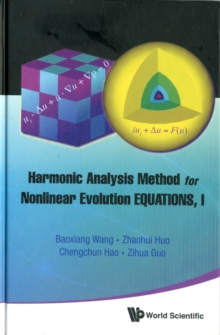Supporting your high street Find out how »
• My AccountHarmonic Analysis Method For Nonlinear Evolution Equations, I Hardback

Description

This monograph provides a comprehensive overview on a class of nonlinear evolution equations, such as nonlinear Schroedinger equations, nonlinear Klein-Gordon equations, KdV equations as well as Navier-Stokes equations and Boltzmann equations.

The global wellposedness to the Cauchy problem for those equations is systematically studied by using the harmonic analysis methods.This book is self-contained and may also be used as an advanced textbook by graduate students in analysis and PDE subjects and even ambitious undergraduate students.

Information

• Format: Hardback
• Pages: 300 pages
• Publisher: World Scientific Publishing Co Pte Ltd
• Publication Date:
• Category: Differential calculus & equations
• ISBN: 9789814360739

£87.00

£70.75

on all orders

Pick up orders

from local bookshops